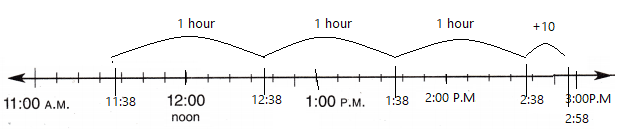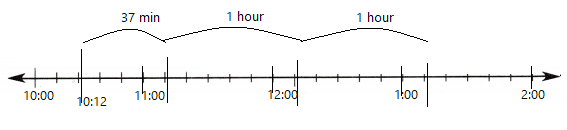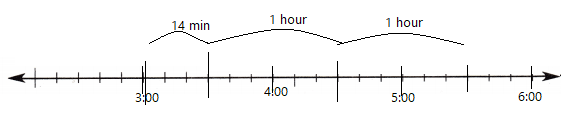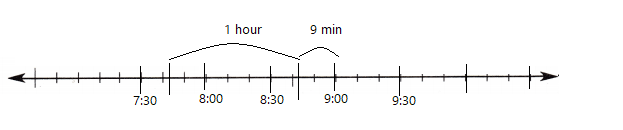Refer to our Texas Go Math Grade 4 Answer Key Pdf to score good marks in the exams. Test yourself by practicing the problems from Texas Go Math Grade 4 Module 16 Assessment Answer Key.

Vocabulary

• balance
• deposit
• second
• withdrawal

Choose the best term from the box to complete the sentence.

Question 1.
A _____________ is a small unit of time.
Explanation:
1 hour = 3600 seconds

Question 2.
The ____________ is the amount of money that is in an account after a deposit or withdrawal is made.
Explanation:
In banking and accounting, the balance is the amount of money owed (or due) on an account. In bookkeeping, “balance” is the difference between the sum of debit entries and the sum of credit entries entered into an account during a financial period.

Question 3.
When money is  added to the balance of an account it is a ____________ .
Explanation:
A sum of money paid into a bank

When money is subtracted from the balance of an account it is a ____________ .
Explanation:
the withdrawal of money from your account at a bank. bank run – the concerted action of depositors who try to withdraw their money from a bank because they think it will fail. withdrawal – the act of taking out money or other capital.

Concepts and Skills

Complete.Question 5.
5 weeks = _____________ days
5 weeks = 35 days
Explanation:
1 week = 7 days
5 x 7 = 35 days

Question 6.
4 minutes = _____________ seconds
4 minutes = 240 seconds
Explanation:
1 Minute = 60 seconds
4 x 60 = 240 seconds

Question 7.
_____________ months = 4 years
12 months = 1 year
4 x 12 = 48

Question 8.
_____________ hours = 5 days
120 hours = 5 days
Explanation:
1 day = 24 hours
5 x 24 = 120 hours

Question 9.
8 years = _____________ weeks
8 x 52 = 416 weeks
Explanation:
1 year = 52 weeks
8 x 52 = 416 weeks

Question 10.
7 days = _____________ hours
7 days = 168 hours
Explanation:
1 day = 24 hours
7 x 24 = 168

Question 11.
14 days = _____________ weeks
14 days = 2 weeks
Explanation:
1 week = 7 days
14 ÷2 = 7

Question 12.
2 years = _____________ weeks
2 years = weeks
Explanation:
1 year = 52 weeks
2 x 52 = 104 weeks

Use a time line to find the start or end time.

Question 13
Start time: 11:38 A.M.
Elapsed time: 3 hours 10 minutes
End time: _____________Explanation:
The elapsed time is shown on a number line
End time: 2:58 pm

Question 14.
Start time: _____________
Elapsed time: 2 hours 37 minutes
End time: 1:12 P.M.Explanation:
Start time is 10:12 A.M

Start time: _____________
Elapsed time: 2 hours 14 minutes
End time: 5:30 P.M.Explanation:
Start time: 3:00 PM

Question 16.
Start time: 7:41 P.M.
Elapsed time: 1 hour 9 minutes
End time: _____________Explanation:
End time: 9:01 PM

Question 17.
Jerome has a balance of $63.45 in his checking account. He withdraws$38.14 to buy a computer game. What is his checking account balance now?
(A) $35.31 (B)$25.31
(C) $101.59 (D)$5.31
Explanation:
Jerome has a balance of $63.45 in his checking account. He withdraws$38.14 to buy a computer game.
63.45 – 38.14 = 25.31
His balance is $25.31 Question 18. Serena buys 3 T-shirts at$12 each and 2 pairs of sandals at $24 each. How much does Serena spend? (A)$74
(B) $12 (C)$84
(D) $96 Answer: C Explanation: Serena buys 3 T-shirts at$12 each and
3 x 12 = 36
2 pairs of sandals at $24 each. 2 x 24 = 48 36 + 48 = 84 Serena spend$84

Question 19.
Ken had a balance of $1,150.00 in his checking account. He withdrew$85.35 to buy groceries. One week later he deposited $250.00 into his checking account. What is the balance in his checking account now? TEKS 4.8,C (A)$1,314.65
(B) $900.00 (C)$335.35
(D) $1,335.35 Answer: A Explanation: 1,150.00 – 85.35 = 1064.65 1064.65 + 250.00 = 1,314.65 1,314.65 is the balance in his checking account now Question 20. Vince bought 8 hardback books to read during the summer. He spent$288 for the books. If each book cost the same amount, how much did one book cost?
(A) $58 (B)$17
(C) $36 (D)$125
He spent $288 for the books. 288 ÷ 8 = 36 one book cost is$36